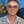0%
0%

# Basic Pulsed Neural Network Simulation

A simulation of a basic pulsed neural network, just slightly different than a traditional neural network.

Similar projects worth following
474 views
A software simulation of a pulsed neural network written in python. It's only a single neuron simulation, with three synapses. It also will plot the output using the matplotlib library.

Currently there are two parts to this project. The first is to write the simulation software, and the second is to write a simple program to plot the outputs with reference to the input times.

• ### The Basic Functions

JangoJungle06/09/2015 at 01:21 0 comments

During my free time in the past few weeks I've worked on my code, so here's a semi-fleshed out skeleton of the code so far. It will also be on Github in the coming weeks. There's a couple of modifications to do, specifically, a better and more thorough plot of not just the neuron pulse times, but also the synaptic event times, and also the projected pulse time calculations need a little tweaking.

```'''
# Neural Network Model
# This program will simulate an event driven model of a pulsed neural network.
'''
__author__ = 'JangoJungle'

from numpy import *
import random as rand
import matplotlib.pyplot as plt

# A quick function to get the synapse state form for plotting  -- Not Used Yet
def get_state(w, t, a):
stateraw = Decimal(w - w * abs(t - a))
index = 0
for x in stateraw:
if x <= 0:
stateraw[index] = 0
index += 1
return stateraw

# declare/initialize variables

# other variables
next_event_time = 0
current_time = 0
fault = 9999999
count = 0
interval = linspace(0,49,50)

# synaptic variables
synaptic_weights = r_[[1, 1, 1]]
state_of_synapses = r_[[0, 0, 0]] # 1 for up, -1 for down, 0 for idle
synaptic_delays = r_[[rand.randint(0, 3), rand.randint(0, 3), rand.randint(0, 3)]]
synaptic_event_times = r_[[1, fault, 1]] # start the trigger and pulse train [pulse train, neuron output, trigger]
synapse_connection_matrix = r_[1, 1, 1]

# neuron variables
previous_activity_slope = r_
current_activity_slope = r_
threshold = 1.5
neuron_pulse_times = r_[fault]
neuron_connection_matrix = c_[[0, 1, 0]] # only one column: only one neuron, connected to synapse 2
pulse_points = linspace(0,0,50)

# Start loop:

while (count < 50):

# Go to next event time

current_time = next_event_time

# Calculate the Next Neuron firing time -- how to adjust this for multiple neurons?
current_activity_slope = sum(state_of_synapses*synaptic_weights) + previous_activity_slope
if current_activity_slope > 0:
neuron_pulse_times = threshold/current_activity_slope + current_time
else:
neuron_pulse_times = fault
previous_activity_slope = current_activity_slope

# Find the next event time and type

next_synapse_event_time = min(synaptic_event_times)
next_synapse = argmin(synaptic_event_times) 	# note: argmin returns a 1d index

next_neuron_firing_time = min(neuron_pulse_times)
next_neuron = argmin(neuron_pulse_times)

next_event_times = r_[[next_synapse_event_time, next_neuron_firing_time]]
next_event_time = min(next_event_times)

event_type = argmin(next_event_times) 	# 0 for synapse, 1 for neuron

# Do required operations for each event type

if next_event_time != fault:
'''
# If it's a synapse event time, update the states
'''
if event_type == 0: 	# synaptic event == 0
# Update states
if state_of_synapses[next_synapse] == 0:
state_of_synapses[next_synapse] = synaptic_weights[next_synapse]
synaptic_event_times[next_synapse] += 1
print ('Synapse {} is firing.'.format(next_synapse))

elif state_of_synapses[next_synapse] == synaptic_weights[next_synapse]:
state_of_synapses[next_synapse] = -synaptic_weights[next_synapse]
synaptic_event_times[next_synapse] += 1

elif state_of_synapses[next_synapse] == -synaptic_weights[next_synapse]:
state_of_synapses[next_synapse] = 0
synaptic_event_times[next_synapse] = fault

# reset the pulse train if it's the pulse train
if next_synapse == 0:
synaptic_event_times[next_synapse] = (2 + current_time + synaptic_delays[next_synapse])

'''
# If it's a neuron firing time, reset the synapses, find all the synapses this neuron is connected to
# and then update those synapses
'''
if event_type == 1: 	# neuron firing = 1
print ('Neuron is firing.')
# reset synapses
state_of_synapses = state_of_synapses = state_of_synapses = 0
synaptic_event_times = synaptic_event_times = synaptic_event_times = fault

# find all the synapses this neuron is connected to and update them
connected_synapses...```

Share

## Discussions

JangoJungle wrote 05/04/2015 at 14:55 point

Thank you! And thanks Bruce Land for the links! Looks like you're heading the direction I want to go with this: Hardware. That's great! Good work!

Are you sure? yes | no

zakqwy wrote 05/03/2015 at 18:28 point

Great project idea!

Are you sure? yes | no

Bruce Land wrote 05/03/2015 at 11:57 point

Are you sure? yes | no

## Similar Projects

Project Owner Contributor

### Honey-bee agent simulation making swarm decisions.Bruce Land

# Does this project spark your interest?

Become a member to follow this project and never miss any updates